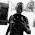JAMB TUTORIAL: PHYICS( GUIDELINES TO MOTION) - EduStuff

## Monday, February 3, 2020

JAMB TUTORIAL  PHYICS (MOTION)

So you saw the topic, motion and you hissed? ,yes you may have been taught motion in school but am not here to waste your time, am giving you deep secrets of motion in exchange of your time.

Edustuff realize that many are not well prepared for this upcoming exam, so we are trying our best to help.

MOTION PART 1️⃣

Motion by definition is the change of position of a body with respect to time.
Motion can also refers to the movement of a body from one direction to another direction with respect to time.

A big question here?

So what causes Motion ?

Pull or push causes the motion of an object. And both are examples of force so it can be easy agreed that force causes Motion

So let's talk about the types of Motion, I know you are eager to learn about big formulas but you first have to understand all this small details.

Types of Motion

1️⃣  Random motion : This is a motion in which an object moves suddenly and rapidly in all directions e.g molecules of a gas which moves in all direction(Zig -zag form).

Another very important example is the movement of footballer on the soccer pitch, they don't stay in one direction.They all move randomly and rapidly following the ball.

2️⃣  Translational Motion : It is a motion in which an object moves from one place to another in a straight line e.g light rays moving in a straight line, movement of humans from one one place to another, movement of cars on the street

3⃣ Oscillatory motion : It  is a motion in which an object swings to and fro between two points e.g the swinging of a pendulum Bob of a wall clock,, The to and fro movement of the testicles of a cow 😹

4⃣ Rotational Motion : This is the motion of a body round a fixed point E.g the rotation of a blade of a suspended ceiling fan, the rotation of the earth round the sun on its axis..

5⃣ Relative Motion :This is not a common type of motion.. This is the motion between two bodies
Is like the measure of the speed of body A in respect to body B

TERMS EXCLUSIVE TO MOTION

1️⃣ Speed : is defined as the rate of change of distance, in other words, speed is the distance moved per unit time, its tells us how fast or slow an object is moving
Speed = distance moved
---------------------
Time taken
In symbols  v= d
---
t
Where v= speed
d= distance
t= time

The unit of speed is m/s or ms-¹, and speed is also a scalar quantity since it has magnitude only.

Examples
Ronaldo ran a distance of 100 metres in 9.83 seconds, what's ronaldo speed?
==================
For every question involving calculations, you must first write your parameters out.
Distance (d)  =100m
time(t)  =9.83s

Speed = Distance
-------------
Time
v =d
----
t
V= 100
-----
9.83

V= 10.2

JUST 10.2???, Then you are very wrong, have you forgotten the unit of speed, the unit is ms-¹
So V =10.2ms-¹

2️⃣ Distance : is the length of an object ,it has no direction e.g 5m

3⃣ Displacement : is the distance measured In a specific direction. It has magnitude and direction e.g 5m due west
Note : both distance and Displacement are measured in mere(m)  or kilometers(km)

4⃣  Velocity : is defined as the rate of Displacement with time
Velocity  = Displacement
--------------------
Time
The unit of velocity is also m/s or ms-¹
Note: tho velocity and speed have the same unit and often interchange, Velocity is a vector quantity while speed is a scalar quantity

5️⃣  Acceleration:is the rate of change of velocity
a= ∆v
----
∆t
a= v - u
-------
t
a = acceleration
u= intital velocity
V= final velocity
t= time taken

Acceleration is also measured in ms-²
Velocity is also measured in ms-¹
While time is measured in secs
So with all those terms explained
Lets  move to some calculated examples

1️⃣
A moving car covers a distance of 10m in 2secs, find the speed?
=====================
Write down the parameters
Distance (d)  = 10m
time (t)  =2s
Speed (v)  =?
Speed = distance
-------------
time
v=d
---
t
V= 10
-----
2
V=5
Only 5?
Have you forgotten the unit of speed /velocity
V=5ms-¹/ read as 5 metre per seconds

2️⃣
A man covers a displacement of 6m in 3 secs.  Calculate the velocity
==========================
Displacement (d)  = 6m
Time =3s
Velocity (v)  =?
Velocity =displacement
--------------------
time
V= d
---
t
V=6
---
3
V=2ms-¹

3⃣
A car accelerates uniformly from 10ms-¹ to 20ms-¹ in 5 seconds, Find its acceleration ?
°========================
acceleration = change in velocity
.             ---------------------------
time
a = v - u
--------
t
u= initial velocity =10ms-¹
v= final velocity =20ms-¹
t=time=5s
a=acceleration =?
a= 20 - 10
-----------
5
a=10
----
5
a=2
Oh!!
Are we not forgetting something here, the unit of acceleration is ms-²
So a=2ms-²

That marks the end of class 1️⃣ of motion ,class 2️⃣ will be coming soon and class 2️⃣ will be based on equations and more calculations on motion.

Hope you enjoyed the class.Kindly use the comment box to say anything .

Leonidas and  EDUSTUFF CEO (MasterMind) wishes you success in the forthcoming examinations.

1.Thumb up for the job well done. May God enlarge the coast of Edustuff. Amen.

1.Amen , Thanks .

2.I love this class, looking forward for class 2 hope my chrome won't disappoint me then thanks mastermind

1.You're welcome . Thanks for following us up.

3.Thanks lot Sir